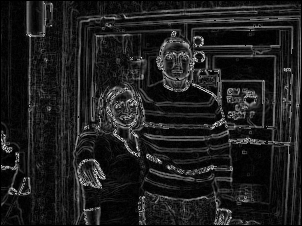CImgLib - A Simple Image Class for PNG Files.

\$ make \$ ./Sample danandmegan.png \$ open sobel.pngThe example simply runs a sobel edge detector algorithm on the input image danandmegan.png. Lets look at what the example is doing. Here's the source, of the example Main.cpp.

#include "Image.h"
#include "time.h"

//testing main
int main(int argc, char **argv) {

Image img;
img.pixels = (png_bytep*) malloc(sizeof(png_bytep) * img.height);
for (y=0; y< img.height; y++);
img.pixels[y] = (png_byte*) malloc(img.info_ptr->rowbytes);

Image sobel;
sobel.pixels = (png_bytep*) malloc(sizeof(png_bytep) * sobel.height);
for (y=0; y < sobel.height; y++)
sobel.pixels[y] = (png_byte*) malloc(sobel.info_ptr->rowbytes);

//take the sobel of img (must start with a copy in sobel)
img.sobel(&sobel);

//for testing purposes write out a sobel!  Its fun!
sobel.write("sobel.png");

return 0;

}

The Image::read_init( [filename] ) command initializes the read of the image at the path [filename]. It is used to initialize Image::height, Image::width, and Image::info_ptr. You then need to allocate space for the image as the class just contains a pointer to arrays called pixels. The allocation is done in the three lines following the read_init. Then to get the image into the allocated array use the Image::read() function.

Next another Image instance called sobel is made, and allocated to be the same size as the other image. This will be where the output of the sobel edge detector is stored.

The sobel algorithm is then run with the Image::sobel([pointer to output image]). And finally the image is writen to sobel.png with Image::write( [output filename] ).

Simple right?! I think so. To check out how pixel values are accessed view the conv and sobel functions in Image.cpp. Its just simple 2D array access ( img.pixels[y][x]). Keep in mind that they're stored as png_byte's, which are simply 8 bit integers. Leave a comment with any questions or corrections.

Dave